# How to Remove Duplicates from Linked List

## How to Remove Duplicates from Linked List in C Language:

In this article, I am going to discuss How to Remove Duplicates from Linked List in C Programming Language with Examples. Please read our previous article, where we discussed How to Check if a Linked List is Sorted in C Language with Examples.

##### How to Remove Duplicates from Linked List:

Here, we will see the procedure for removing duplicate elements from a sorted linked list. Here is an example of a sorted linked list of elements: 4, 4, 7, 7, 7. So ‘4’ and ‘7’ are duplicate elements.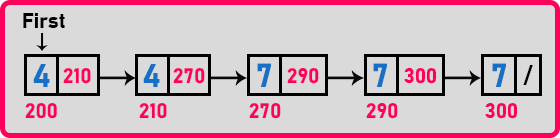We want to remove duplicates from the list. What should be the procedure for removing duplicates from a LinkedList? Let us understand the procedure for removing the elements.

##### Procedure to Remove Duplicates from Linked List:

We need two pointers. Let us take one pointer ‘p’ on the first node and ‘q’ be the pointer on the next node.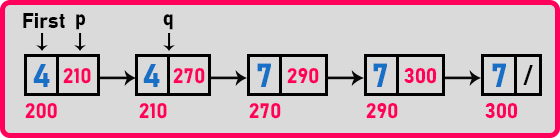The idea is we have to check whether the current node and the next node data are the same. If the data is the same, we can delete one of the nodes. So let us delete the second node. So, for that, we need two pointers.

The procedure is to check whether the data in the p’s node and the q’s node is matching or not. In this case, p and q data are matching. If the data is matching then we have to delete the second node. For deleting a node, make the previous node point to the current node’s next as shown in the below image.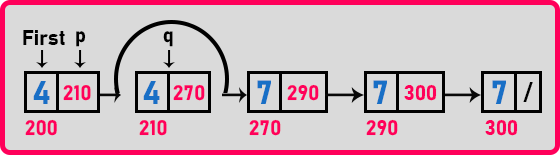Then delete the node where ‘q’ is pointing,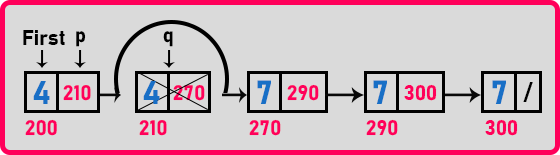Now make ‘q’ points on ‘q = q->next’,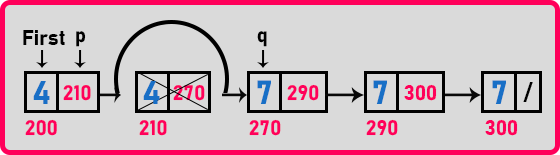In this, we have performed deletion of the duplicate nodes. Now check the ‘p’ and ‘q’ data. Is it the same? No, ‘p’ and ‘q’ are different. So, move ‘p’ to ‘q’ and ‘q’ to ‘q->next’ as follows.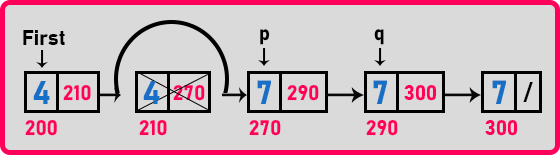Again, p’s and q’s data are the same. So here we will perform deletion of q’s node as we performed in the previous step. After deletion of all the duplicate nodes, the linked list is,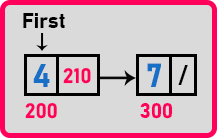Now let us write the pseudo-code.

##### Pseudo Code for Remove Duplicates from Linked List:

Node *p = first;
Node *q = first->next;
while(q != NULL){
if(p->data != q->data){
p = q;
q = q->next;
}
else{
p->next = q->next;
delete q;
q = p->next;
}
}

Now let us look at the complete program.

##### Program for Remove Duplicates from Linked List using C Language:
```#include <stdio.h>
#include <stdlib.h>

struct Node
{
int data;
struct Node *next;
} *first = NULL;

void Create(int A[], int n)
{
int i;
struct Node *t, *last;
first = (struct Node *) malloc (sizeof (struct Node));
first->data = A;
first->next = NULL;
last = first;

for (i = 1; i < n; i++)
{
t = (struct Node *) malloc (sizeof (struct Node));
t->data = A[i];
t->next = NULL;
last->next = t;
last = t;
}
}

void Display(struct Node *p)
{
while (p != NULL)
{
printf ("%d ", p->data);
p = p->next;
}
}

void RemoveDuplicate(struct Node *p)
{
struct Node *q = p->next;

while (q != NULL)
{
if (p->data != q->data)
{
p = q;
q = q->next;
}
else
{
p->next = q->next;
free(q);
q = p->next;
}
}
}

int main()
{
int A[] = { 4, 4, 7, 7, 7 };
Create (A, 5);

printf ("Linked List with Duplicates: \n");
Display (first);

RemoveDuplicate (first);

printf ("\nLinked List without Duplicates: \n");
Display (first);
return 0;
}
```
###### Output: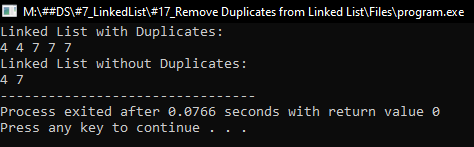In the next article, I am going to discuss How to Reverse a Linked List in C Language with Examples. Here, in this article, I try to explain How to Remove Duplicates from Linked List in C Language with Examples and I hope you enjoy this article.